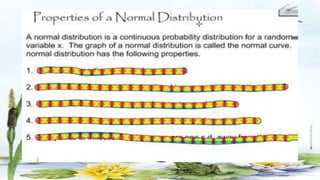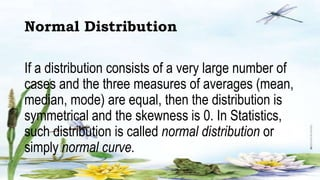Anzeige1 von 22
Anzeige

### NORMAL DISTRIBUTION.pptx

1. A PLEASANT MORNING TO EVERYONE !!!
2. LET US BE UNITED Form a figure out of the pieces of the puzzle provided. (2 mins.)
3. QUESTIONS: •What have you formed? Say something about the figure. •Is there similar graphs?
4. •If we are to group the graphs/figures you formed, which should be together? •How do these figures differ from each other?
5. OBJECTIVES: •Determine / enumerate the characteristics of a normal distribution. •Sketch / construct a normal curve which represents a normal distribution. •Cite real-life examples involving normal distribution.
6. Normal Distribution If a distribution consists of a very large number of cases and the three measures of averages (mean, median, mode) are equal, then the distribution is symmetrical and the skewness is 0. In Statistics, such distribution is called normal distribution or simply normal curve.
7. The normal curve has a very important role in inferential statistics. It provides a graphical representation of statistical values that are needed in describing the characteristics of populations as well as in making decisions. It is defined by an equation that uses the populations mean, and the standard deviation. There is no single curve, but rather a whole family of normal curves have the same
8. The normal probability distribution has the following properties: 1. The distribution curve is bell-shaped.
9. 2. The curve is symmetrical about its center. 3. The mean, the median, and the mode coincide at the center. 4. The width of the curve is determined by the standard deviation of the distribution.
10. 5. The tails of the curve flatten out indefinitely along the horizontal axis, always approaching the axis but never touching it. That is, the curve is asymptotic to the base line. 6. The area under the curve is 1. Thus, it represents the probability or proportion or the percentage associated with specific sets of measurement values.
11. APPLICATION: Cite some examples in real-life where you can see the normal curve or distribution.
12. Student’s Average Report
13. Shoe Size
14. Birth Weight
15. Income Distribution in Economy
16. Technical Stock Market
17. GENERALIZATION: What are the properties of the normal distribution?
18. EVALUATION: Modified True or False If the statement is true or check, write the letter T, but if the statement is wrong then change the underlined word to make the statement right. 1. The standard normal distribution is also called normal curve. 2. The area under a normal curve is 100.
19. 3. The mean of a standard normal curve is 3. 4. The curve of a normal distribution extends indefinitely at the tails. 5. The shape of the normal probability distribution is symmetric about the mean.
20. AGREEMENT Study about the lesson.

### Hinweis der Redaktion

1. In this group activity, each should should have a collaboration with each member. From the tiltle itself “Let us be united” it requires unity and teaming up with one another.
2. Coincide – occupy the same space. And that certain space is at the center of the curve. Symmetrical refers to having both halves of the graph equal.
3. The normal birth weight of a newborn range from 2.5 to 3.5 kg.
4. The income of a country lies in the hands of enduring politics and government. It depends upon them how they distribute the income among the rich and the poor community. We all are well aware of the fact that the middle-class population is a bit higher than the rich and poor population. So, the wages of the middle-class population makes the mean in the normal distribution curve.
Anzeige